Generalized Maximum Entropy for Multinomial Discrete Choice Models

Multinomial discrete choice models take the form of an experiment that consists oftrials. On each trial, one ofalternatives is observed. If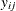is the random variable that takes on the value 1 when alternativeis selected for theth trial and 0 otherwise, then the probability thatis 1, conditional on a vector of regressors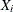and unknown parameter vector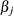, is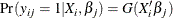where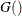is a link function. For noisy data the model becomes: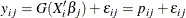The standard maximum likelihood approach for multinomial logit is equivalent to the maximum entropy solution for discrete choice models. The generalized maximum entropy approach avoids an assumption of the form of the link function.

The generalized maximum entropy for discrete choice models (GME-D) is written in primal form as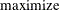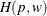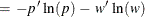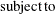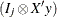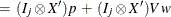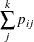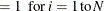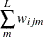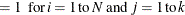Golan, Judge, and Miller (1996) have shown that the dual unconstrained formulation of the GME-D can be viewed as a general class of logit models. Additionally, as the sample size increases, the solution of the dual problem approaches the maximum likelihood solution. Because of these characteristics, only the dual approach is available for the GME-D estimation method.

The parametersare the Lagrange multipliers of the constraints. The covariance matrix of the parameter estimates is computed as the inverse of the Hessian of the dual form of the objective function.